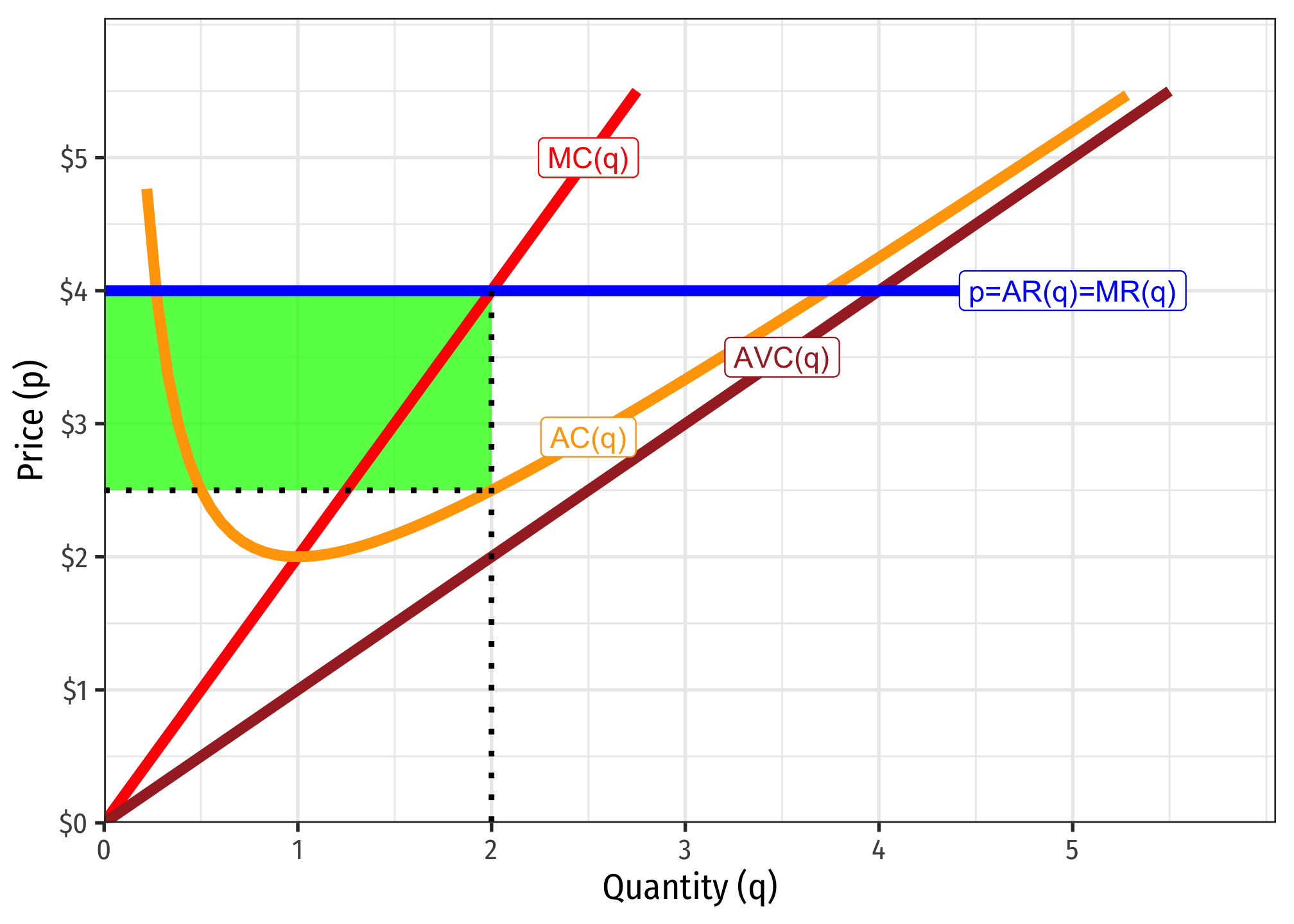A firm has short-run costs given by: \begin{aligned} C(q)&=q^2+1\\ MC(q)&=2q\\ \end{aligned}

Fixed costs are the part of the total cost function that do not change with output. Imagine if $$q=0$$ and the firm produced nothing, it would still have to pay 1. $f=1$ ### 2. Write an equation for variable costs, $$VC(q)$$. These are the terms of the cost function that change with output (have a variable in them). $VC(q)=q^2$ ### 3. Write an equation for average fixed costs, $$AFC(q)$$. $AFC(q)=\frac{C(q)}{q}=\frac{1}{q}$ ### 4. Write an equation for average variable costs, $$AVC(q)$$. $AVC(q)=\frac{VC(q)}{q}=\frac{q^2}{q} = q$ ### 5. Write an equation for average (total) costs, $$AC(q)$$. $AC(q)=\frac{C(q)}{q}=\frac{q^2+1}{q}=q+\frac{1}{q}$ Alternatively: \begin{align*} AC(q)&=AVC(q)+AFC(q)\\ AC(q)&=q+\frac{1}{q}\\ \end{align*} ### 6. Suppose the firm is in a competitive market, and the current market price is4, how many units of output maximize profits?

The firm’s profit maximizing quantity of output occurs where $$MR(q)=MC(q)$$. Since we know for a competitive firm, $$MR(q)$$ is the same is the price, we can set $$p=MC(q)$$ to solve for $$q^*$$:

\begin{align*} p=MR&=MC\\ 4&=2q\\ q^*&=2 \\ \end{align*}

### 7. How much profit will this firm earn?

Profit is total revenues minus total costs:

\begin{align*} \pi &= R(q)-C(q)\\ \pi &= pq-(q^2+1) \\ \pi &= (4)(2)-(2^2 + 1) \\ \pi &= (8)-(5)\\ \pi &= \3\\ \end{align*}

Total profits are 3. We could also calculate this using price and average cost: \begin{align*} \pi &=q(p-AC(q))\\ \pi &=q(p-\big[q+\frac{1}{q}\big])\\ \pi &=2(4-\big[2+\frac{1}{2}\big])\\ \pi &=2(4-[2.50])\\ \pi &=2(1.50)\\ \pi&=\3\\ \end{align*}We can see visually that profits (in green) are the area of the box between price4.00 and average cost at $$q^*=2$$, which is $2.50. Thus, profit per unit is$1.50, for two units, a total profit of \$3.00.

### 8. At what market price would the firm break even $$(\pi=0)$$?

A firm breaks even where profits are zero, and we know that is where price equals average cost. We want to find the lowest possible average cost, as that is the price that would produce no profits. Since price is marginal revenue, and the firm always sets $$MR(q)=MC(q)$$, we know that the minimum of the average cost curve is where it is equal to marginal cost, so we set:

\begin{aligned} AC(q)&=MC(q)\\ q+\frac{1}{q}&=2q \\ q^2+1&=2q^2 \\ 1&=q^2 \\ 1&=q^* \\ \end{aligned}

We know the quantity but we need to find the price where the firm breaks even, so plugging this back into either marginal cost or average cost:

\begin{aligned} MC(q)&=2q\\ MC(1)=2(1)\\ MC(1)&=2\\ \end{aligned}

\begin{aligned} AC(q)&=q+\frac{1}{q}\\ AC(1)=(1)+\frac{1}{(1)}\\ AC(1)&=2\\ \end{aligned}